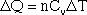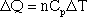JEE 2006 Sample Question Paper : Page 1 | Page 2 | Page 3 | Page 4 | Page 5 | Page 6 | Page 7

# IIT JEE 2006 Sample Question Paper

Passage 1

When heat is added to a gas, the temperature increases and the gas expands. If the gas is in a rigid container, then the volume remains constant and the pressure increases. If the gas in in a container that can expand (for example a cylinder with a vertical piston), then the pressure remains constant but the volume expands.

The amount of energy required to increase the temperature of one mole of gas is called the specific heat; there is a specific heat for constant pressure (Cp) and a specific heat for constant volume ((Cv).

In equation form, this is written:Delta Q is the energy required to raise the temperature of the gas by a certain amount (delta T). The specific heats for a few gases, including monoatomic gases such as helium and argon, and diatomic gases such as nitrogen and oxygen, are given in the table below.

 Specific Heat of Some Gases Gas Cv Cp Cp-Cv Helium(H) 3.00 4.98 1.98 Argon(A) 3.00 5.00 2.00 Nitrogen(N2) 4.96 6.95 1.99 Oxygen(O2) 4.96 6.95 1.99 Carbon Monoxide(CO) 4.93 6.95 2.02 Carbon Dioxide(CO2) 6.74 8.75 2.01 Methane(CH2) 6.48 8.49 2.01 Note: All quanties are in calories(Kelvin*mole)

Q. 1 Let X be the energy needed to raise the temperature of 5 moles of nitrogen held at constant pressure by one degree. Let Y be the energy needed to raise 5 moles of carbon monoxide by one degree with the pressure held constant. What is the ratio X:Y?

1. 5:7
2. 1:1
3. 7:5
4. 7:9

Discription: There is no need to do any calculations here. If you put X on top of Y, you will see that it reduces to the ratio of Cp for nitrogen over Cp for carbon monoxide. And since both gases have the same Cp, the ratio is simply 1:1.

Q. 2 The difference Cp - Cv is a constant. This constant is often called R, the universal gas constant. Which of the following is true given the data?

1. For a monoatomic gas, Cp = 3/2 R
2. For a diatomic gas, Cp = 3/2 R
3. For a monoatomic gas, Cv = 3/2 R
4. For a diatomic gas, Cv = 3/2 R

Discription: Helium and argon are monoatomic gases, and we can see that Cv = 3/2 *R = (3/2)*2 = 3.

Q. 3 How much energy would be required to heat two moles of methane by one degree if the gas is kept at constant volume?

1. 6.5 calories
2. 8.5 calories
3. 11 calories
4. 13 calories

Description: The definition of Cv is the amount of energy required to heat one mole of a gas by one degree. Therefore, to heat two moles of methane by one degree will require 2*6.5 = 13 calories.

Q. 4 Which of the following is a possible explanation for the fact that Cp is always greater than Cv?

1. Some of the energy is used to expand the container in order to maintain constant pressure.
2. A rigid container does not conduct heat as well as one that can change shape.
3. There are generally more moles of gas when the pressure is kept constant than when the volume is kept constant.
4. There are generally fewer moles of gas when the pressure is kept constant than when the volume is kep constant.

Discription: Because Cp is always greater than Cv we know that it takes more energy to increase a given amount of gas when the pressure is held constant. It is reasonable that the extra energy is used to increase the volume of the container.

Q. 5 A certain amount of energy, X, is sufficient to raise the temperature of 60 moles of argon by T degrees when the pressure is constant. How many moles of argon can be raised by T degrees with the same amount of energy X, if the volume is held constant?

1. 30
2. 50
3. 75
4. 100

Discussion: You can use the two equations:You are given that X = 60*5*T. Let n be the number of moles that can be heated to temperature T with X calories of energy. Then X = n*3*T, and for this equation to be consistent with the previous one, it must be that n = 100.IIT JEE - HomeWebsites of IITJEE SyllabusIIT Question PapersBooks For IITIIT JEE 2008IIT JEE 2007IIT JEE 2006IIT JEE 2005IIT CoursesSeats ReservationEligibility CriteriaAnalysis of IIT JEEIIT AlumniIIT JEE TipsIIT Articles